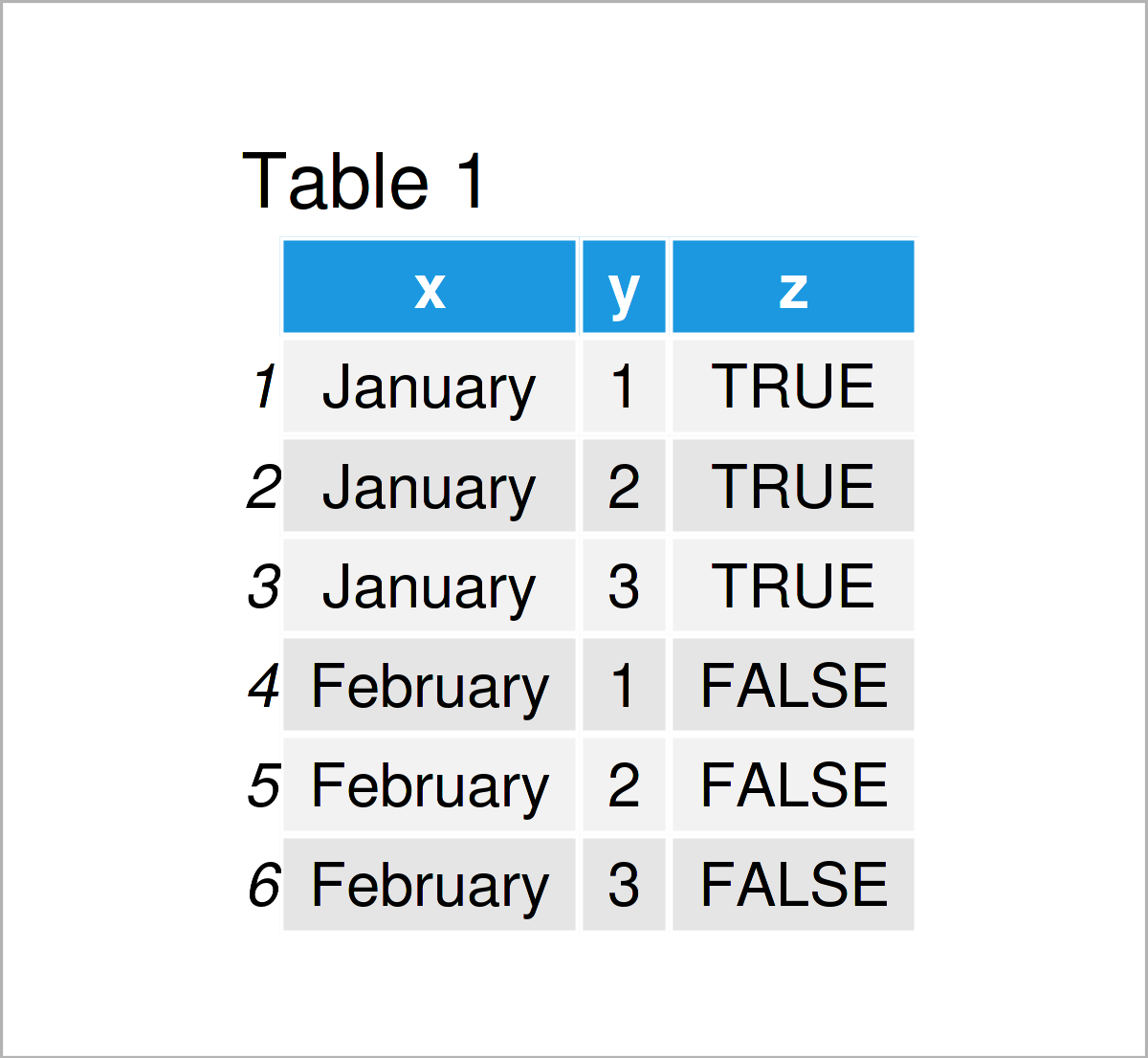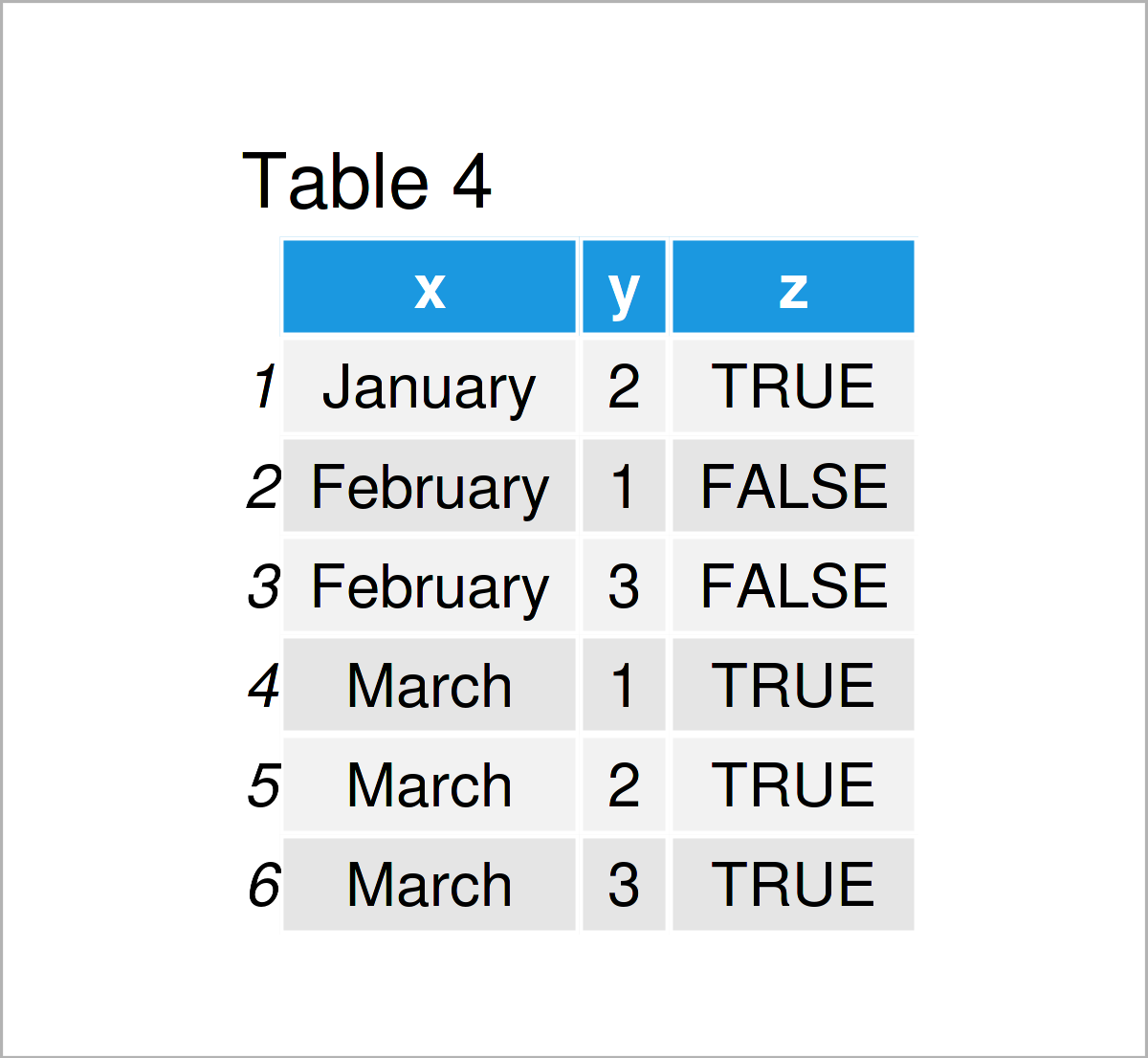# Filter Rows of data.table in R (3 Examples)

This post demonstrates how to filter the rows of a data.table in the R programming language.

Let’s just jump right in.

## Example Data & Packages

First, we have to install and load the data.table package:

```install.packages("data.table")                                # Install data.table package

We also create some example data.

```dt_all <- data.table(x = rep(month.name[1:3],     each = 3),
y = rep(c(1, 2, 3),           times = 3),
z = rep(c(TRUE, FALSE, TRUE), each = 3))  # Create data.tableTable 1 shows the top six rows of the example data – You can see that our data consists of three columns.f

## Example 1: Filter Rows by Column Values

In this example, I’ll demonstrate how to select all those rows of the example data for which column x is equal to February. With the use of %in%, we can choose a set of values of x. In this example, the set only contains one value.

`dt_all[x %in% month.name[c(2)], ]                             # Rows where x is February`After executing the previous R syntax, the data is reduced to those rows for which variable x is equal to February, shown in Table 2.

## Example 2: Filter Rows by Multiple Column Value

In the previous example, we addressed those rows of the example data for which one column was equal to some value. In this example, we condition on the values of multiple columns.

`dt_all[x %in% month.name[c(2)] & y == 1, ]                    # Rows, where x is February and y is 1`By running the previous syntax, we have created Table 3, i.e. we display those rows of the example data for which variable x is February and variable y is equal to 1.

## Example 3: Remove Rows by Index Number

Sometimes, we do not want to select rows for which certain logical conditions hold, but rather choose all those rows for which certain conditions do not hold. The following syntax shows how to delete rows by index. It is like the inverse argument of selecting certain rows.

```dt_some_removed <- dt_all[ - c(1,3,5), ]                      # Remove rows number 1, 3, and 5By running the previous syntax, we have created Table 4, i.e. data.table dt_all, where rows 1, 3, and 5 were removed.

## Video, Further Resources & Summary

Do you need further info on the examples of this article? Then I recommend having a look at the following video on my YouTube channel. In the video, I explain the R programming syntax of this article in the R programming language.

In addition, you may want to have a look at the other posts on my website. A selection of tutorials can be found below:

Summary: This article has shown how to get the rows of a data.table to which certain column values apply in R. If you have additional questions, please let me know in the comments below. Furthermore, please subscribe to my email newsletter to get updates on new tutorials.

This page was created in collaboration with Anna-Lena Wölwer. Have a look at Anna-Lena’s author page to get further information about her academic background and the other articles she has written for Statistics Globe.

Subscribe to the Statistics Globe Newsletter